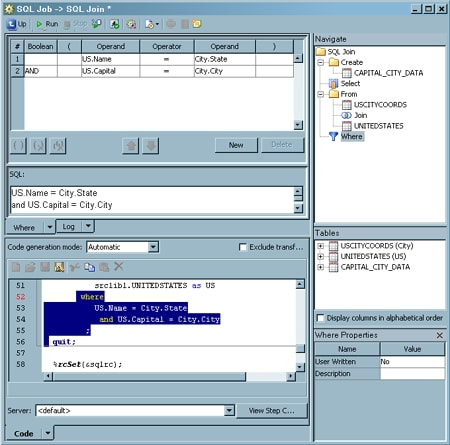# Creating or Configuring a WHERE Clause

## Problem

You want to configure the WHERE clause for an SQL query that is used in a SAS Data Integration Studio job. The conditions included in this clause determine which subset of the data from the source tables is included in the query results that are collected in the target table.

## Solution

You can use the Where tab in the Designer window for the SQL Join transformation to configure the WHERE clause for an SQL query.

### Configure the WHERE Clause with the Where Tab

The WHERE clause for the query is an SQL expression that creates subsets of the source tables in the SQL query. It also defines the join criteria for joining the source tables and the subquery to each other by specifying which values to match. Perform the following steps to configure the Where tab:
1. If the Where clause object is missing from the process flow in the Diagram tab, double-click Where in the SQL Clauses pane. The Where clause object is added to the query flow in the Diagram tab. Note that Where clause objects are automatically populated into the Diagram tab. The WHERE clause is not automatically generated under the following circumstances:
• the query contains only one source table
• no relationship was found during the auto-join process
2. Click Where in the Navigate pane to access the Where tab.
3. Click New on the Where tab to begin the first condition of the expression. An editable row appears in the table near the top of the tab.
4. Enter the appropriate operands and operator for the first condition.
5. Add the remaining conditions for the WHERE clause. You need to add one row for each condition.
6. The conditions created for the sample query are depicted in the SQL code that is generated in this step in the SQL field, as shown in the following display.
Sample Where Tab SettingsNote that the SQL code for the WHERE clause that is shown in the SQL field is identical to the highlighted WHERE clause code that is displayed on the Code tab. To highlight the code for a query object such as the Where object, right-click the object in the Navigate pane and click Find In. Then, click Code in the submenu.
Note that WHERE conditions are not optimized for these types of conditions:
• arithmetic operators
• variable-to-variable condition
• sounds-like operator
• any function other than SUBSTR and TRIM